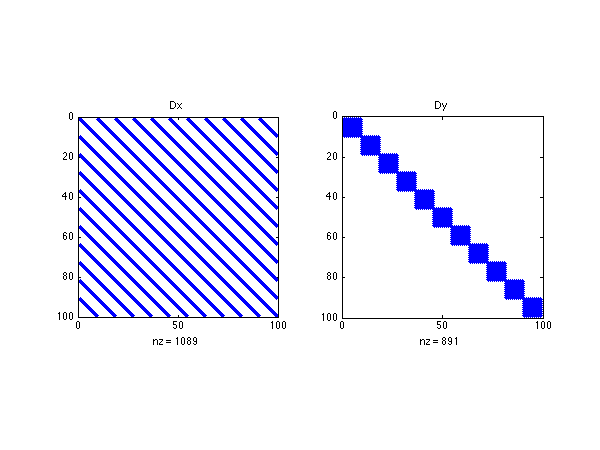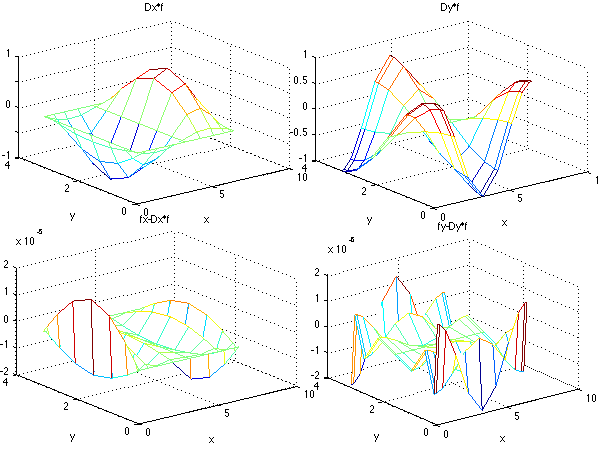# sandbox/easystab/diffmat_2D.m

(We do the same but in 3D in diffmat_3D.m)

In this code, we show and test the differentiation matrices for a 2D domain, that is, an unknown function that depends on two parameters x and y. For this we use just the same kind of differentiation matrices that we had for 1D problems, but we combine them together. Just as for 1D, the function is discretized, not on a 1D mesh, but on a 2D mesh. So the 2D function u(x,y) becomes \displaystyle u(j,i)=u(x_i,y_j).

Please note that the first index in the array u correspond to y and the second corresponds to x. It is best to do so because of the way 2D functions are ploted in Octave/Matlab.

In order to adapt all the things we did until now for this 2D functions, we will transform u which naturally is a rectangular array into a long column vector. Then all the linear operations will be again matrix-vector multiplications. The figure below shows the transformation from an array into a vector. It simply ammounts to stacking on top of each others the successive columns of the array representation:Sketch of the array-vector transformations

The transformation from array to column vector is coded

uvec=u(:);

and the backward transformation from column vector to array is coded

u=reshape(uvec,Ny,Nx);

because the array has Ny lines and Nx columns. These are the first operations for the 2D functions.

clear all; clf

%%%% parameters and flags
Nx=11; % gridpoints in x
Ny=9; % gridpoints in x
Lx=2*pi % domain size in x
Ly=pi % domain size in y


# 1D matrices

Please see diffmat_dif1D.m for a description of how to build the 1D differentiation matrices. We build the dx and dy with different sizes and number of points. Here we use differentiatino matrices based Chebychev polynomials.

%1D differentiation matrices
[dx,dxx,wx,x]=dif1D('cheb',0,Lx,Nx,3);
[dy,dyy,wy,y]=dif1D('cheb',0,Ly,Ny,3);


# 2D matrices

Now the question is: how should we transform the 1D differentiation matrices in a manner compatible with the vector representation of the 2D variables? This is simple to figure out for the y derivative, see the figure below:y differentiation matrix for a 2D mesh

The D.y matrix is a big block-diagonal matrix whose diagonal elements are copies of the 1D d.y differentiation matrix. For the x derivative it is a litle less intuitive. Please meditate upon the figure below:x differentiation matrix for a 2D mesh

Each block of D.x is a diagonal matrix with as diagonal elements the copies of one of the elements of the 1D d.x matrix.

Once figured out, it is very quick to code that using the kronecker product. The Kronecker multiplication of matrices ammounts to stacking next to each other many copies of the same matrix. Here is the answer for help kron:

KRON Kronecker tensor product. KRON(X,Y) is the Kronecker tensor product of X and Y. The result is a large matrix formed by taking all possible products between the elements of X and those of Y. For example, if X is 2 by 3, then KRON(X,Y) is

  [ X(1,1)*Y  X(1,2)*Y  X(1,3)*Y
X(2,1)*Y  X(2,2)*Y  X(2,3)*Y ]

We buid Dx and Dy out of dx and dy and with identity matrices of the good size. Remember that Octave is case-sensitive, that is Dcan be a different variable from d. We had already the 1D meshes x and y, and we now as well build mesh arrays using the function meshgrid. This functino does something close to kronand is very convenient for building arrays from functions of xand y, in a much easier way than one may think at first. Here too, Xand Y are the 2D equivalents of xand y.

These operations are coded also in the function dif2D.m.diffmat_2D_dif2D.m is just like the present code but using dif2D.m instead of building explicitely the matrices. Also dif2D.m builds for you the integration weights for a 2D grid.

% 2D differentiation matrices
Dx=kron(dx,eye(Ny));
Dy=kron(eye(Nx),dy);


# Meshgrid

For plotting the 2D functions and also to build the matrices for mathematical functions it is very convenient to build an array representation of the mesh. Indeed x is a vector and y is a vector, but u is an array. This is done by the function meshgrid who works as follows:Illustration of the output of the Octave/Matlab function meshgrid

The help meshgrid gives:

MESHGRID   X and Y arrays for 3-D plots.
[X,Y] = MESHGRID(x,y) transforms the domain specified by vectors
x and y into arrays X and Y that can be used for the evaluation
of functions of two variables and 3-D surface plots.
The rows of the output array X are copies of the vector x and
the columns of the output array Y are copies of the vector y.

[X,Y] = MESHGRID(x) is an abbreviation for [X,Y] = MESHGRID(x,x).
[X,Y,Z] = MESHGRID(x,y,z) produces 3-D arrays that can be used to
evaluate functions of three variables and 3-D volumetric plots.

For example, to evaluate the function  x*exp(-x^2-y^2) over the
range  -2 < x < 2,  -2 < y < 2,

[X,Y] = meshgrid(-2:.2:2, -2:.2:2);
Z = X .* exp(-X.^2 - Y.^2);
surf(X,Y,Z)
[X,Y]=meshgrid(x,y);


# Test

To test our differentiation matrices, we will compare the analytical and numerical derivatives for trigonometric functions. You see here how convenient is meshgrid. Be aware here in uilding f fx and fythat we use the .*multiplication which is the element by element multiplication, as opposed to the *multiplication which by default is the matrix multiplication.

% Analytical derivatives
f=cos(X).*sin(Y);
fx=-sin(X).*sin(Y);
fy=cos(X).*cos(Y);


# Numerical derivative

The derivative are simply obtained by multiplication of the vector representation of f. This representation is obtained by saying that we want all the elements of the array f. This is a shortcut notation to transform an aray into a vector. Then we do a reshape in order to come back to the array representation for the plotting.

% Nuerical derivatives
fX=reshape(Dx*f(:),Ny,Nx);
fY=reshape(Dy*f(:),Ny,Nx);


# Structure of the matrices

Using the spy command we display in a figure the sparsity structure of the two differentiation matrices. We see that they are very different as sketched in the figure above. Since Chebychev differentation matrices in 1D are full, the Dy is block diagonal with dy stacked on the diagonal, whereas Dx is banded.

% showing the structure of Dx and Dy
figure(1)
subplot(1,2,1); spy(Dx); title('Dx');
subplot(1,2,2); spy(Dy); title('Dy');The structure of the matrices

# Comparison

We show the shape of the numerical derivatives and the erreor between the exact derivative and the numerical derivative. We have chosen to take very few gridpoints because otherwise the error would be close to machine accuracy.

% results
figure(2)
subplot(2,2,1);mesh(X,Y,fX); xlabel('x'); ylabel('y'); title('Dx*f');
subplot(2,2,2);mesh(X,Y,fY); xlabel('x'); ylabel('y'); title('Dy*f');
subplot(2,2,3);mesh(X,Y,fx-fX); xlabel('x'); ylabel('y'); title('fx-Dx*f');
subplot(2,2,4);mesh(X,Y,fy-fY); xlabel('x'); ylabel('y'); title('fy-Dy*f');The comparison of exact and numerical derivatives